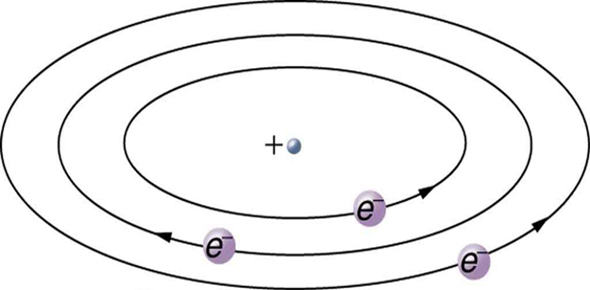# Circuits

8 QuestionsSettingsSee how good you are at answering questions on Series and Parallel Circuits, as well as on Voltage and Current.

Related Topics
• 1.
In Physics, the dumbest student is...?
• 2.
What circuit symbol is this?
• A.

A Bulb

• B.

A Motor

• C.

A Fuse

• D.

A Cell

• 3.
Voltage does not split in a parallel circuit.
• A.

True

• B.

False

• 4.
In a Series circit, the current...?
• A.

Stays the same

• B.

Splits at a branch

• C.

Is given to the cell

• D.

Is shared amongst components

• 5.
A single battery is called a ----?
• 6.
Current is the amount of energy provided by the circuit, increasing this made the electrons travel faster.
• A.

True

• B.

False

• 7.
Resistance is moving electrons are stopped by large positive ---- in a circuit to produce this.
• 8.
This is the symbol for:
• A.

An Ammeter

• B.

A Voltmeter

• C.

A Buzzer

• D.

An Electric Shock